rayshader.com Go back Open original

# Create and Visualize Hillshaded Maps from Elevation Matrices

rayshader is an open source R package for producing 2D and 3D hillshaded maps of elevation matrices using a combination of raytracing, spherical texture mapping, overlays, and ambient occlusion. It also includes the ability to export 3D models to a 3D print-ready format, and includes a post-processing depth of field effect for 3D visualizations.

``````# To install the latest version from Github:
# install.packages("devtools")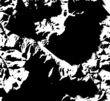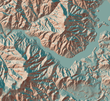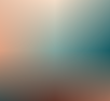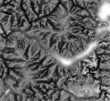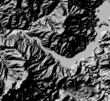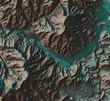• `ray_shade` uses user specified light directions to calculate a global shadow map for an elevation matrix. By default, this also scales the light intensity at each point by the dot product of the mean ray direction and the surface normal (also implemented in function `lamb_shade`, this can be turned off by setting `lambert=FALSE`.
• `sphere_shade` maps an RGB texture to a hillshade by spherical mapping. A texture can be generated with the `create_texture` function, or loaded from an image. `sphere_shade` also includes 7 built-in palettes: “imhof1”,“imhof2”,“imhof3”,imhof4“,”desert“,”bw“,”unicorn“.
• `create_texture` programmatically creates texture maps given five colors: a highlight, a shadow, a left fill light, a right fill light, and a center color for flat areas. The user can also optionally specify the colors at the corners, but `create_texture` will interpolate those if they aren’t given.
• `ambient_shade` creates an ambient occlusion shadow layer, darkening areas that have less scattered light from the atmosphere. This results in valleys being darker than flat areas and ridges.
• `lamb_shade` uses a single user specified light direction to calculate a local shadow map based on the dot product between the surface normal and the light direction for an elevation matrix.
• `add_shadow` takes two of the shadow maps above and combines them, scaling the second one (or, if the second is an RGB array, the matrix) as specified by the user.
• `add_overlay` takes a 3 or 4-layer RGB/RGBA array and overlays it on the current map. If the map includes transparency, this is taken into account when overlaying the image. Otherwise, the user can specify a single color that will be marked as completely transparent, or set the full overlay as partly transparent.

Rayshader also has three functions to detect and add water to maps:

• `detect_water` uses a flood-fill algorithm to detect bodies of water of a user-specified minimum area.
• `add_water` uses the output of `detect_water` to add a water color to the map. The user can input their own color, or pass the name of one of the pre-defined palettes from `sphere_shade` to get a matching hue.
• `render_water` adds a 3D tranparent water layer to 3D maps, after the rgl device has already been created. This can either add to a map that does not already have a water layer, or replace an existing water layer on the map.

• `render_depth` generates a depth of field effect for the 3D map. The user can specify the focal distance, focal length, and f-stop of the camera, as well as aperture shape and bokeh intensity. This either plots the image to the local device, or saves it to a file if given a filename.
• `render_label` adds a text label to the `x` and `y` coordinate of the map at a specified altitude `z` (in units of the matrix). The altitude can either be specified relative to the elevation at that point (the default), or absolutely.

And four functions to display and save your maps:

• `plot_map` Plots the current map. Accepts either a matrix or an array.
• `write_png` Writes the current map to disk with a user-specified filename.
• `plot_3d` Creates a 3D map, given a texture and an elevation matrix. You can customize the appearance of the map, as well as add a user-defined water level.
• `render_snapshot` Saves an image of the current 3D view to disk (if given a filename), or plots the 3D view to the current device (useful for including images in R Markdown files).

All of these functions are designed to be used with the magrittr pipe `%>%`.

``````library(rayshader) #Here, I load a map with the raster package.
elmat = matrix(raster::extract(localtif,raster::extent(localtif),buffer=1000), nrow=ncol(localtif),ncol=nrow(localtif)) #We use another one of rayshader's built-in textures:
elmat %>%
plot_map()````````````#sphere_shade can shift the sun direction:
elmat %>%
sphere_shade(sunangle = 45, texture = "desert") %>%
plot_map()``````Rayshader also supports 3D mapping by passing a texture map (either external or one produced by rayshader) into the `plot_3d` function.

``````elmat %>%
plot_3d(elmat,zscale=10,fov=0,theta=135,zoom=0.75,phi=45, windowsize = c(1000,800))
render_snapshot()``````You can also easily add a water layer by setting `water = TRUE` in `plot_3d()` (and setting `waterdepth` if the water level is not 0), or by using the function `render_water()` after the 3D map has been rendered. You can customize the appearance and transparancy of the water layer via function arguments. Here’s an example using bathymetric/topographic data of Monterey Bay, CA (included with rayshader):

``````montshadow = ray_shade(montereybay,zscale=50,lambert=FALSE)
montereybay %>%
plot_3d(montereybay,zscale=50,fov=0,theta=-45,phi=45,windowsize=c(1000,800),zoom=0.75, water=TRUE, waterdepth = 0, wateralpha = 0.5,watercolor = "lightblue", waterlinecolor = "white",waterlinealpha = 0.5)
render_snapshot()``````Rayshader also has map shapes other than rectangular included `c("hex", "circle")`, and you can customize the map into any shape you want by setting the areas you do not want to display to `NA`.

``````par(mfrow=c(1,2))
montereybay %>%
plot_3d(montereybay,zscale=50,fov=0,theta=-45,phi=45,windowsize=c(1000,800),zoom=0.6, water=TRUE, waterdepth = 0, wateralpha = 0.5,watercolor = "lightblue", waterlinecolor = "white",waterlinealpha = 0.5,baseshape = "circle")
render_snapshot()
rgl::rgl.clear() montereybay %>%
plot_3d(montereybay,zscale=50,fov=0,theta=-45,phi=45,windowsize=c(1000,800),zoom=0.6, water=TRUE, waterdepth = 0, wateralpha = 0.5,watercolor = "lightblue", waterlinecolor = "white",waterlinealpha = 0.5,baseshape = "hex")
render_snapshot()``````Adding text labels is done with the `render_label()` function, which also allows you to customize the line type, color, and size along with the font:

``````montereybay %>%
plot_3d(montereybay,zscale=50,fov=0,theta=-100,phi=30,windowsize=c(1000,800),zoom=0.6, water=TRUE, waterdepth = 0, waterlinecolor = "white", waterlinealpha = 0.5, wateralpha = 0.5,watercolor = "lightblue")
render_label(montereybay,x=350,y=240, z=4000,zscale=50, text = "Moss Landing",textsize = 2,linewidth = 5)
render_label(montereybay,x=220,y=330, z=6000,zscale=50, text = "Santa Cruz",color="darkred",textcolor = "darkred",textsize = 2,linewidth = 5)
render_label(montereybay,x=300,y=130, z=4000,zscale=50, text = "Monterey",dashed = TRUE,textsize = 2,linewidth = 5)
render_label(montereybay,x=50,y=130, z=1000,zscale=50, text = "Monterey Canyon",relativez=FALSE,textsize = 2,linewidth = 5)
render_snapshot()``````You can also apply a post-processing effect to the 3D maps to render maps with depth of field with the `render_depth()` function:

``````elmat %>%